1. /
2. CBSE
3. /
4. Class 07
5. /
6. Mathematics
7. /
8. NCERT Solutions for Class...

# NCERT Solutions for Class 7 Maths Exercise 14.1### myCBSEguide App

Download the app to get CBSE Sample Papers 2023-24, NCERT Solutions (Revised), Most Important Questions, Previous Year Question Bank, Mock Tests, and Detailed Notes.

NCERT solutions for Maths Symmetry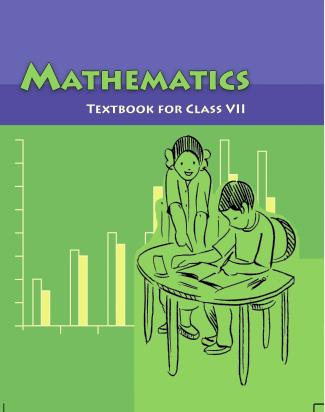## NCERT Solutions for Class 7 Maths Symmetry

###### Question 1. Copy the figures with punched holes and find the axes of symmetry for the following:

(a)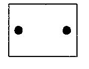(b)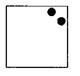(c)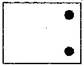(d)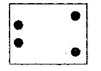(e)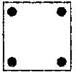(f)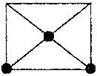(g)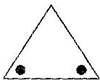(h)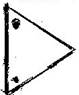(i)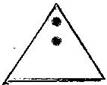(j)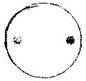(k)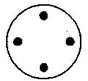(l)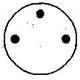S.No. Punched holed figures The axes of symmetry (a)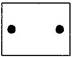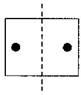(rectangle) (b)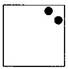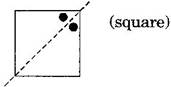(c)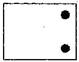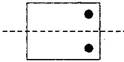(d)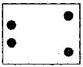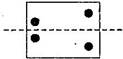(e)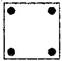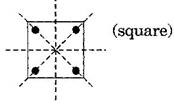(f)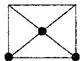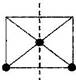(g)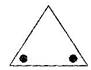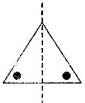(h)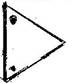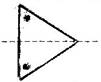(i)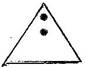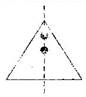(j)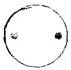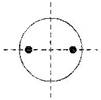(k)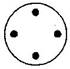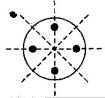(l)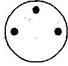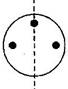###### Question 2. Given the line(s) of symmetry, find the other hole(s):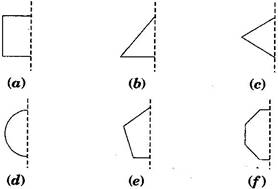S.No. Line(s) of symmetry Other holes on figures (a)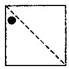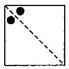(b)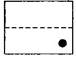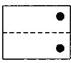(c)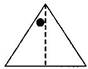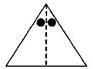(d)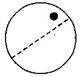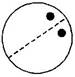(e)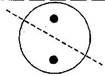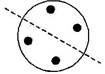###### Question 3. In the following figures, the mirror line (i.e., the line of symmetry) is given as a dotted line. Complete each figure performing reflection in the dotted (mirror) line. (You might perhaps place a mirror along the dotted line and look into the mirror for the image).

Are you able to recall the name of the figure you complete?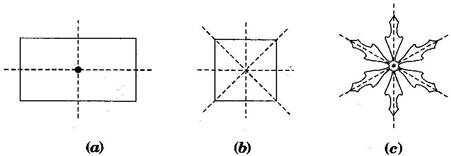S.No. Question figures Complete figures Names of the figure (a)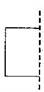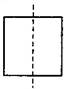Square (b)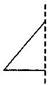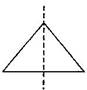Triangle (c)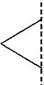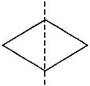Rhombus (d)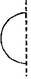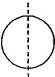Circle (e)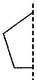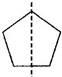Pentagon (f)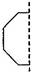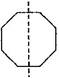Octagon

NCERT Solutions for Class 7 Maths Exercise 14.1

###### Question 4. The following figures have more than one line of symmetry. Such figures are said to have multiple lines of symmetry: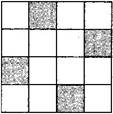Identify multiple lines of symmetry, if any, in each of the following figures:

 S.No. Problem Figures Lines of symmetry (a)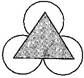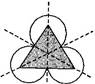(b)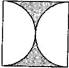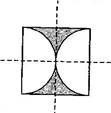(c)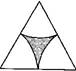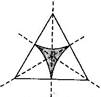(d)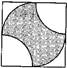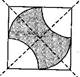(e)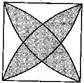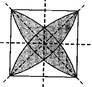(f)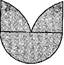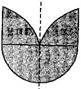(g)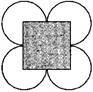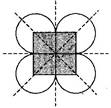(h)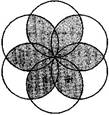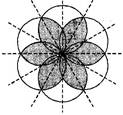NCERT Solutions for Class 7 Maths Exercise 14.1

###### Question 5. Copy the figure given here: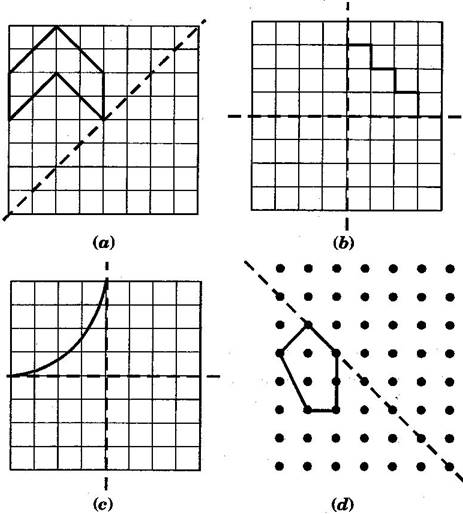Take any one diagonal as a line of symmetry and shade a few more squares to make the figure symmetric about a diagonal. Is there more than one way to do that? Will the figure be symmetric about both the diagonals?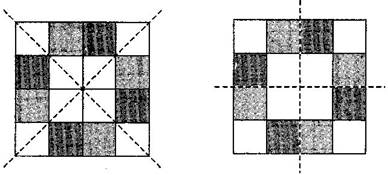Yes, there is more than one way.

Yes, this figure will be symmetric about both the diagonals.

NCERT Solutions for Class 7 Maths Exercise 14.1

###### Question 6. Copy the diagram and complete each shape to be symmetric about the mirror line(s):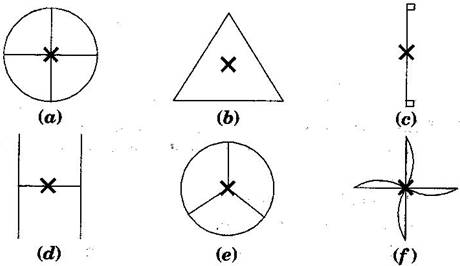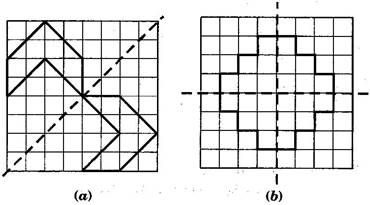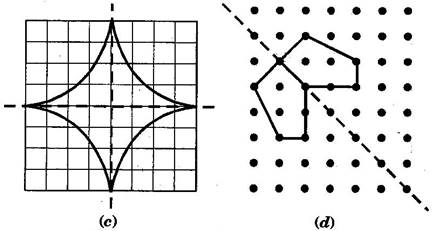NCERT Solutions for Class 7 Maths Exercise 14.1

###### Question 7. State the number of lines of symmetry for the following figures:

(a) An equilateral triangle(b) An isosceles triangle(c) A scalene triangle

(d) A square(e) A rectangle(f) A rhombus

(g) A parallelogram(h) A quadrilateral(i) A regular hexagon

(j) A circle

 S.No. Figure’s name Diagram with symmetry Number of lines (a) Equilateral triangle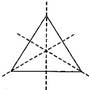3 (b) Isosceles triangle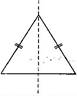1 (c) Scalene triangle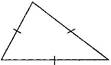0 (d) Square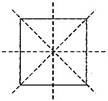4 (e) Rectangle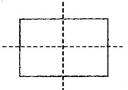2 (f) Rhombus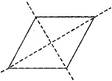2 (g) Parallelogram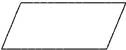0 (h) Quadrilateral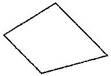0 (i) Regular Hexagon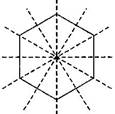6 (j) Circle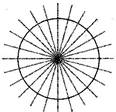Infinite

NCERT Solutions for Class 7 Maths Exercise 14.1

###### Question 8. What letters of the English alphabet have reflectional symmetry (i.e., symmetry related to mirror reflection) about:
1. a vertical mirror
2. a horizontal mirror
3. both horizontal and vertical mirrors
1. Answer: (a) Vertical mirror – A, H, I, M, O, T, U, V, W, X and Y

mirror mirror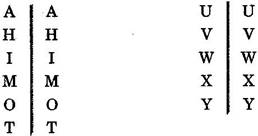(b) Horizontal mirror – B, C, D, E, H, I, O and X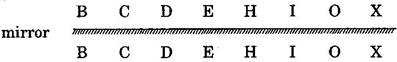(c) Both horizontal and vertical mirror – H, I, O and X

Question 9. Give three examples of shapes with no line of symmetry.

2. Scalene triangle
3. Parallelogram

NCERT Solutions for Class 7 Maths Exercise 14.1

###### Question 10. What other name can you give to the line of symmetry of:
1. an isosceles triangle?
2. a circle?

Answer: (a) The line of symmetry of an isosceles triangle is median or altitude.

(b) The line of symmetry of a circle is diameter.

## NCERT Solutions for Class 7 Maths Exercise 14.1

NCERT Solutions Class 7 Maths PDF (Download) Free from myCBSEguide app and myCBSEguide website. Ncert solution class 7 Maths includes text book solutions from Class 7 Maths Book . NCERT Solutions for CBSE Class 7 Maths have total 15 chapters. 7 Maths NCERT Solutions in PDF for free Download on our website. Ncert Maths class 7 solutions PDF and Maths ncert class 7 PDF solutions with latest modifications and as per the latest CBSE syllabus are only available in myCBSEguide.

## CBSE app for Students

To download NCERT Solutions for Class 7 Maths, Social Science Computer Science, Home Science, Hindi English, Maths Science do check myCBSEguide app or website. myCBSEguide provides sample papers with solution, test papers for chapter-wise practice, NCERT solutions, NCERT Exemplar solutions, quick revision notes for ready reference, CBSE guess papers and CBSE important question papers. Sample Paper all are made available through the best app for CBSE students and myCBSEguide website.### Test Generator

Create question paper PDF and online tests with your own name & logo in minutes.### myCBSEguide

Question Bank, Mock Tests, Exam Papers, NCERT Solutions, Sample Papers, Notes

1. Very good

2. thank you

3. Nice

4. Thats good

5. Wow!